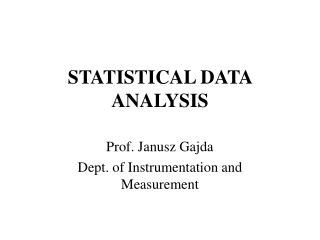DownloadDownload PresentationSTATISTICAL DATA ANALYSIS

# STATISTICAL DATA ANALYSIS

Download Presentation## STATISTICAL DATA ANALYSIS

- - - - - - - - - - - - - - - - - - - - - - - - - - - E N D - - - - - - - - - - - - - - - - - - - - - - - - - - -
##### Presentation Transcript

1. STATISTICAL DATA ANALYSIS Prof. Janusz Gajda Dept. of Instrumentation and Measurement

2. Plan of the lecture • Classification of the measuring signals according to their statistical properties. • Definition of basic parameters and characteristics of the stochastic signals (expected value, variance, mean-square value, probability density function, power spectral density, joined probability density function, cross-correlation function, spectral density function, transfer function).

3. Interpretation of the basic statistical characteristics. • Elements of statistic: estimation theory and hypothesis • verification theory, parametric and non-parametric • estimation, point and interval estimation. • Good properties of the estimator: • unbiased, • effective, • zgodny, • robust.

4. Application of the point estimation and interval • estimation methods in determination of estimates • of these parameters and characteristics. • Estimators of basic parameters and characteristics • of random signals: mean value and variance, • probability density function, autocorrelation and • cross correlation function, power spectral density and • mutual spectral density, coherency function, transmittance. • Analysis of the statistical properties of those estimators. • Determination of the confidence intervals of basic • statistical parameters for assumed confidence level. • Statistical hypothesis and their verification. • Errors of the first and second order observed during • the verification process.

5. Classification of the measuring signals according to their statistical properties

6. Periodic signals Mono-harmonic signals: Where: - signal amplitude, - angular frequency, - initial phase angle,

7. Poly-harmonic signals: Where: - amplitude of the n-th harmonic component, - basic angular frequency, - initial phase angle of the n-th component,

8. Frequency spectrum of the periodic signals - is the measurable number

9. Classification of the measuring signals according to their statistical properties

10. Set of realizations of the random quantity

11. Basic statistical characteristics Mean-square value: Root-mean-square value

12. Expected value Variance:

13. Probability function: Probability density function:

14. Most popular distributions: Standardised normal distribution:

15. Most popular distributions: Normal distribution:

16. Normal distribution – cumulative probability

17. Normal distribution - kwantyle

18. Most popular distributions: Chi-square distribution( ) - number of freedom degrees

19. Chi-square distribution – cumulative distribution

20. Most popular distributions: t- Student distribution – probability density: f – number of freedom degrees

21. t - Student distribution – cumulative probability:

22. Auto-correlation function.

23. Power spectral density:

24. Joined density of probability function:

25. Joined cumulative probability:

26. Cross – correlation:

27. Spectral density function:

28. Transfer function:

29. Statistical Base of Data Analysis Mathematical statistics deals with gaining information from data. In practice, data often contain some randomness or uncertainty. Statistics handles such data using methods of probability theory. Mathematical statistics tests the distribution of the random quantities

30. In applying statistics to a scientific, industrial, or societal problem, one begins with a process or population to be studied. This might be a population of people in a country, of crystal grains in a rock, or of goods manufactured by a particular factory during a given period. It may instead be a process observed at various times; data collected about this kind of "population" constitute what is called a time series. For practical reasons, one usually studies a chosen subset of the population, called a sample. Data are collected about the sample in an observational or measurement experiment. The data are then subjected to statistical analysis, which serves two related purposes: description and inference.

31. Descriptive statistics can be used to summarize the data, • either numerically or graphically, to describe the sample. • Basic examples of numerical descriptors include • the mean and standard deviation. • Graphical summarizations include various kinds of charts • and graphs. • Inferential statistics is used to model patterns in the data, • accounting for randomness and drawing inferences about the • larger population. These inferences may take the form of answers • to yes/no questions (hypothesis testing), estimates of numerical • characteristics (estimation), descriptions of association (correlation) • or modelling of relationships (regression).

32. A statistical hypothesis test, or more briefly, hypothesis test, • is an algorithm to state the alternative (for or against the hypothesis) • which minimizes certain risks. • The only conclusion, which may be draw-out from the test isthat • There is not enough evidence to reject the hypothesis. • Hypothesis is false.

33. Estimation theory is a branch of statistics and that deals with estimating the values of parameters based on measured/empirical data. The parameters describe the physical object that answers a question posed by the estimator.

34. Nonparametric estimation is a statistical method that allows determination of the chosen characteristic, understood as a set of points in predefined coordinates system (without any functional description).